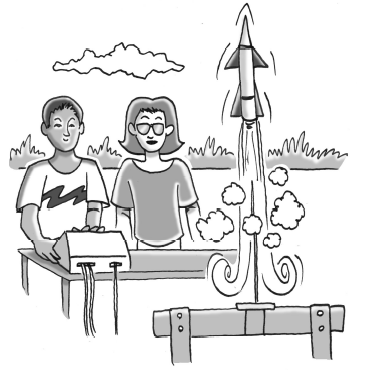Home > CCAA8 > Chapter cca9 > Lesson cca9.2.1 > Problem9-54

9-54.

Robbie builds model rockets. One day he sets up a rocket, backs away from the launch pad, and then shoots the rocket off into the air. The rocket’s path is represented by the equation $y=−10x^2+130x−400$, where $y$ is the height in meters off the ground and $x$ is the horizontal distance in meters from Robbie. 9-54 HW eTool (Desmos). Homework Help ✎

1. Use either the Zero Product Property or the Quadratic Formula to find the $x$-intercepts of the path of Robbie’s rocket. What do the $x$-intercepts tell you?

$x=8$ or $x=5$

Coordinates: $(5,0)$ and $(8,0)$

Robbie backed up $5$ m from the launch pad.
The rocket landed $8$ m away from him.perty or the Quadratic Formula to find the $x$-intercepts of the path of Robbie's rocket. What do the $x$-intercepts tell you?

2. When Robbie's rocket lands, how far is it from the launch pad?

The launch pad was 5m from Robbie, and the rocket landed 8m from him in the same direction.Use the graph in the eTool below for this problem.
Click on the link at right for the full eTool version: CCA 9-54 HW eTool